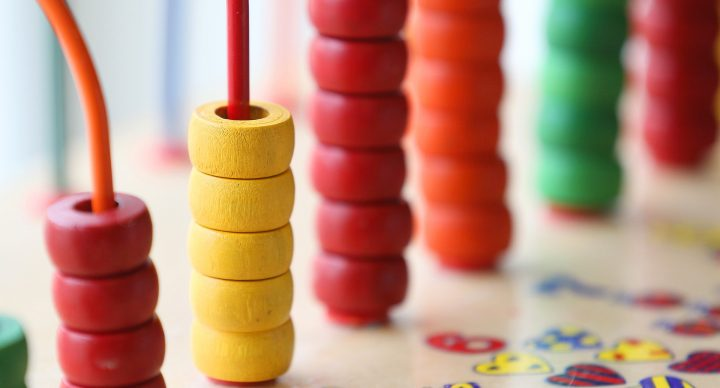### Course Overview

The Acellus Grade 4 Math course examines whole numbers and decimals through processes of composition and decomposition, as well as converting decimals to fractions. It also discusses some of the various forms of addition and subtraction, whether with whole numbers, decimals, and fractions. There are lessons in data representation, money, two dimensional shapes, and measurements, which allows the course to be an exciting and informative experience. Acellus Grade 4 Math is taught by Acellus Instructor Mark Rogers.

### Sample Lesson - Divisibility RulesThis course was developed by the International Academy of Science. Learn More

### Scope and Sequence

Unit 1: Place Value of Whole Numbers As the course is introduced, students will begin by examining digits, values, and periods of whole numbers. Next, they will cover composing and decomposing numbers with place values and expanded notation. Finally, learners will explore rounding and its applications. Unit 2: Place Value of Decimals In this unit learners will start with diving into the digits, values, and periods of decimal numbers, followed by composing and decomposing decimal numbers using various models. Next, they will examine expanded notations and place value with decimals. Students will work with number lines in tenths and hundredths. Unit 3: Decimals and Fraction Comparison In unit 3, students will learn to change decimal tenths and hundredths into fractions and where to place them on a number line. Next, they will explore modeling decimal and fraction mixed numbers. Learners will complete this unit by comparing numbers in a data table and decimal models. Unit 4: Addition and Subtraction This unit begins with showing students the standard algorithms for addition and subtraction with regrouping. After this, they will explore addition and subtraction with rounding and decimal addition and subtraction using coins. Finally, they will dive deeper into decimal addition and subtracting by using regrouping and numbers greater than one. Unit 5: Products In this unit, learners will begin by multiplying by tens and hundreds. After this, they will learn to multiply two, three, and four-digit numbers by one-digit numbers. Next, students will explore two-digit by two-digit multiplication and multiples of ten. Lastly, students will examine equations and two-step multiplication scenarios. Unit 6: Division Unit 6 begins by representing quotients with arrays and area models, followed by divisibility rules for 2, 3, 5, 9, and 10. Next, students will study factoring and dive into prime and composite numbers. After this, they will cover standard algorithms for division and learn to interpret division with remainders. They will end the unit by exploring two-step division scenarios and checking quotients for reasonableness. Unit 7: Unknown Quantities This unit begins by exploring variables as unknown quantities. After this, they will learn to represent sums, differences, products, and quotients with equations. Learners will complete the lesson by discovering multi-step operations with strip diagrams and equations. Unit 8: Sequences and Tables In unit eight, students will begin by examining numeric sequences and then exploring addition, multiplication, subtraction, and division sequences in tables. The last half of this unit will have learners extending these sequences to solve for the nth term. Unit 9: Comparing Fractions This unit begins with learners composing and decomposing fractions. In the next few segments, they will explore equivalent fractions using pictorial models and with multiples. Students will complete the unit by examining denominators and by comparing fractions with different numerators and denominators. Unit 10: Operations on Fractions Unit 10 starts with students discovering benchmarks of fractions and adding fractions with common denominators. Next, they will explore mixed numbers and fractions with sums greater than 1, subtracting fractions with common denominators, and adding and subtracting mixed numbers. Learners will complete the unit by being introduced to word problems featuring fractions and multiplying fractions by whole numbers. Unit 11: Visual Representations of Data In this unit, students will discover frequency tables and using them to solve problems. Following this, they will explore dot plots and stem-and-leaf plots. The unit completes with learners solving two-step problems with frequency tables, dot plots, and stem-and-leaf plots. Unit 12: Constructing and Measuring Angles This unit begins with students discovering points, lines, line segments, rays, and angles. After this, they will examine angles as parts of a circle and how to draw angles with a protractor. Next, learners will be introduced to measuring angles with a protractor and adding angles. They will complete the unit by learning to find a missing angle and exploring parallel and perpendicular lines. Unit 13: 2D Shapes Unit 13 starts with students examining lines of symmetry and classifying triangles. Following this, they will discover polygons with parallel and perpendicular lines as well as with various angles. They will finish the unit by finding the perimeters of rectangles and squares. Unit 14: Measurement This unit begins by exploring the relative sizes of length, mass, and volume using customary and metric measurements. Next, students will learn to convert customary and metric units of length, mass and volume using tables. Finally, they will study measurement applications in length, mass, and volume. Unit 15: Money and Time As the course completes, students will learn to convert hours to minutes and minutes to seconds. Following this, they will explore elapsed time using number lines and how to measure times. As the unit transitions to exploring money, learners will study change after a payment followed by sales, expenses, and profit. They will wrap the course with discovering fixed and variable expenses, saving options, and financial institutions.

This unit is followed by the Final Review and Exam.
This course does not have any sections.